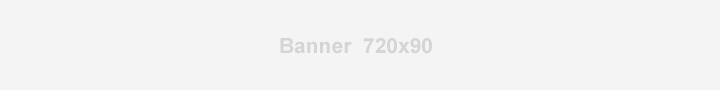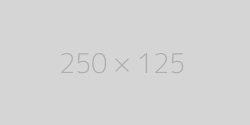## Hex to RGB Color Converter

Enter 6 digits hex color code and press the Convert button:# Hex to RGB Color Converter: Understanding Color Representation in Digital Designs

A Hex to RGB Color Converter is a tool used to convert a color specified in hexadecimal format to its equivalent RGB (Red, Green, Blue) representation. RGB is a color model used in digital displays and is based on mixing different intensities of red, green, and blue light to create a range of colors.

## What is a Hexadecimal Color Representation?

In the hexadecimal format, a color is represented as a 6-digit string, starting with a hash symbol "#". Each pair of digits corresponds to the intensity of red, green, and blue, respectively, in the color. The value of each pair of digits can range from 00 to FF, where FF represents the maximum intensity and 00 represents the minimum intensity.

### How to Convert Hexadecimal to RGB?

To convert a hexadecimal color to RGB, each pair of digits is first converted to its decimal equivalent. This decimal number is then used to calculate the intensity of each color component, with the formula: intensity = decimal value / 255. The resulting values represent the intensities of red, green, and blue, respectively, in the RGB color model.

Example: To convert the hexadecimal color "#FF9933" to RGB, first convert each pair of digits to its decimal equivalent: FF = 255, 99 = 153, 33 = 51. Then, calculate the intensities of each color component using the formula: red = 255/255 = 1, green = 153/255 = 0.6, blue = 51/255 = 0.2. The RGB representation of the color "#FF9933" is (1, 0.6, 0.2).

Overall, a Hex to RGB Color Converter is a useful tool for web developers and graphic designers, as it allows them to easily convert colors between different color models, and to experiment with different color combinations in their designs.